# Nonparametric Spearman’s Correlation for Survival Data

## Introduction

This vignette shows how to use package survSpearman to nonparametrically estimate Spearman’s correlation between two time-to-event variables. It also demonstrates some basic tools for visualizing bivariate survival data.

## Notations and setup

Time-to-event variables are denoted as $$(T_X, T_Y)$$ defined on $$[0, \infty) \times [0, \infty)$$ and time-to-censoring as $$(C_X, C_Y)$$. Time to event or censoring is denoted as $$(X, Y) = (\min(T_X,C_X), \min(T_Y, C_Y))$$, and event indicators as $$\Delta_X = {1}(T_X \leq C_X)$$, $$\Delta_Y = {1}(T_Y \leq C_Y)$$ with $$\delta_X$$ and $$\delta_Y$$ being their realizations. We denote the maximum follow-up times for $$T_X$$ and $$T_Y$$ as $$\tau_X$$ and $$\tau_Y$$, respectively, with no events observed beyond the region $$\Omega = [0, \tau_X) \times [0, \tau_Y)$$, or equivalently, $$C_X \le \tau_X$$ and $$C_Y \le \tau_Y$$. We denote marginal and joint survival functions as $$S_X(x) = \Pr\left(T_X > x \right)$$, $$S_Y(y) = \Pr\left( T_Y > y \right)$$, $$S(x,y) = \Pr\left(T_X > x, T_Y > y \right)$$.

knitr::opts_chunk$set( collapse = TRUE, comment = "#>" ) library(survSpearman) data(data123) ## Examples of computing Spearman’s correlation ### Example 1 In this example, we use simulated data with $$T_X$$ and $$T_Y$$ independently censored before or at $$\tau_X=\tau_Y=2$$ (bivariate generalized type-I censoring with the follow-up time 2 for both variables). data1 = data123[1:100,] data1[1:7, ] #> X deltaX Y deltaY #> 1 0.30857708 1 0.24910560 1 #> 2 0.46541242 1 0.35874902 0 #> 3 0.85062791 1 1.29663538 1 #> 4 0.08668864 0 0.07969093 1 #> 5 0.22524818 1 0.21715157 1 #> 6 2.00000000 0 2.00000000 0 #> 7 1.67677439 0 0.37548431 0 Let’s visualize the data. visualBivarTimeToEvent(X = data1$X, Y = data1$Y, deltaX = data1$deltaX, deltaY = data1$deltaY, xlim = c(0, 3), ylim = c(0, 3), labelX = "X", labelY = "Y", segLength = 0.1, dotSize = 0.3, scaleLegendGap = 1.1, legendCex = 0.6, labCex = 0.6, axisCex = 0.5)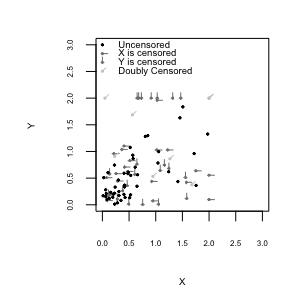Now let’s compute the correlation, res = survSpearman(X = data1$X, Y = data1$Y, deltaX = data1$deltaX, deltaY = data1$deltaY) res[["Correlation"]] #> HighestRank Restricted #> 0.5140414 0.6231143 Function survSpearman() first computes Dabrowska’s bivariate survival surface from time to event data and then uses it to calculate the correlation. This function can also compute correlation directly from the bivariate survival surface, dabrSurf = survDabrowska(data1$X, data1$Y, data1$deltaX, data1$deltaY)$DabrowskaEst
res = survSpearman(bivarSurf = dabrSurf, tauX = 2, tauY = 2)
res[["Correlation"]]
#> HighestRank  Restricted
#>   0.5140414   0.6231143

Because of type-I censoring, the survival probability cannot be fully estimated outside of $$\Omega = [0, \tau_X) \times [0, \tau_Y) = [0, 2) \times [0, 2)$$, so when computing correlation, it makes sense to choose a restricted region with the same follow-up time, $$\Omega_R = [0, 2) \times [0, 2)$$. The function returns the user-specified restricted region as well as the effective restricted region, which are the values just before the latest observed event times,

dabrSurf = survDabrowska(data1$X, data1$Y, data1$deltaX, data1$deltaY)$DabrowskaEst res = survSpearman(bivarSurf = dabrSurf, tauX = 2, tauY = 2) res[["Restricted region set by user"]] #> tauX tauY #> "2" "2" res[["Effective restricted region"]] #> tauX tauY #> "1.97478951013018 +" "1.96005790068168 +" When computing correlation under generalized type-I censoring, we can either assume the highest rank for points outside of the restricted region and compute $$\widehat{\rho}_S^H$$ or compute correlation conditional on being in the restricted region, $$\widehat{\rho}_{S|\Omega_R}$$. Because at least one observation was censored outside of $$[0, 2) \times [0, 2)$$, the probability of being inside the restricted region is less than one, and therefore $$\widehat{\rho}_S^H$$ is not equal to $$\widehat{\rho}_{S|\Omega_R}$$, res[["Correlation"]] #> HighestRank Restricted #> 0.5140414 0.6231143 One could choose values $$\tau_X$$ and $$\tau_Y$$ that are less than those used to generate the data. For example, if the analyst chooses $$\tau_X=\tau_Y = 1.5$$, the function returns the following estimates: res = survSpearman(bivarSurf = dabrSurf, tauX = 1.5, tauY = 1.5) res #>$Restricted region set by user
#>  tauX  tauY
#> "1.5" "1.5"
#>
#> $Effective restricted region #> tauX tauY #> "1.46898672937274 +" "1.32696096438289 +" #> #>$Correlation
#> HighestRank  Restricted
#>   0.5309905   0.5810781

The user can also choose a restricted region that contains $$[0, 2) \times [0, 2)$$, for example $$[0, 3) \times [0, 3)$$, but this is not advisable because there are no events outside of $$[0, 2) \times [0, 2)$$ so setting a larger restricted region will result in the same correlation values as for $$[0, 2) \times [0, 2)$$,

res =  survSpearman(bivarSurf = dabrSurf, tauX = 3, tauY = 3)
res
#> $Restricted region set by user #> tauX tauY #> "3" "3" #> #>$Effective restricted region
#>                 tauX                 tauY
#> "1.97478951013018 +" "1.96005790068168 +"
#>
#> $Correlation #> HighestRank Restricted #> 0.5140414 0.6231143 ### Example 2 In this example, the data are generated with unrestricted follow-up time (unbounded censoring). In this case, $$\widehat{\rho}_S^H$$ is consistent for the overall Spearman’s correlation. If the largest $$X$$ and $$Y$$ correspond to events ($$\delta_X=\delta_Y=1$$), data2 = data123[101:200,] data2[data2$X == max(data2$X), c("X", "deltaX")] #> X deltaX #> 118 4.816644 1 data2[data2$Y == max(data2$Y), c("Y", "deltaY")] #> Y deltaY #> 118 3.683655 1 then the survival surface is fully estimated, and if the restricted region of interest contains the data support, then $$\widehat{S}^H(x,y) =\widehat{S}(x,y|\Omega_R) = \widehat{S}(x,y)$$ and $$\widehat{\rho}_S^H = \widehat{\rho}_{S|\Omega_R}$$, dabrSurf = survDabrowska(data2$X, data2$Y, data2$deltaX, data2$deltaY)$DabrowskaEst
res = survSpearman(bivarSurf = dabrSurf, tauX = Inf, tauY = Inf)
res
#> $Restricted region set by user #> tauX tauY #> "Inf" "Inf" #> #>$Effective restricted region
#>                 tauX                 tauY
#> "4.81664394874279 +" "3.68365466198858 +"
#>
#> $Correlation #> HighestRank Restricted #> 0.5226419 0.5226419 In contrast, if the largest $$X$$ and/or $$Y$$ correspond to censoring events ($$\delta_X$$ and/or $$\delta_Y=0$$), data2[data2$X == max(data2$X), "deltaX"] = 0 then the survival surface cannot be estimated after the last censored event, and therefore $$\widehat{\rho}_S^H$$ is not equal to $$\widehat{\rho}_{S|\Omega_R}$$: dabrSurf = survDabrowska(data2$X, data2$Y, data2$deltaX, data2$deltaY)$DabrowskaEst
res = survSpearman(bivarSurf = dabrSurf, tauX = Inf, tauY = Inf)
res
#> $Restricted region set by user #> tauX tauY #> "Inf" "Inf" #> #>$Effective restricted region
#>                 tauX                 tauY
#> "2.72884335472806 +" "3.68365466198858 +"
#>
#> $Correlation #> HighestRank Restricted #> 0.5226419 0.5028863 ### Example 3 Note that if there is no censoring and $$\tau_X=\tau_Y=\infty$$, then both estimates are equivalent to Spearman’s rank correlation, data3 = data123[201:300,] dabrSurf = survDabrowska(X = data3$X, Y = data3$Y, deltaX = data3$deltaX, deltaY = data3$deltaY)$DabrowskaEst
res = survSpearman(bivarSurf = dabrSurf, tauX = Inf, tauY = Inf)
res
#> $Restricted region set by user #> tauX tauY #> "Inf" "Inf" #> #>$Effective restricted region
#>                 tauX                 tauY
#> "4.50100438256196 +" "4.44359130618219 +"
#>
#> $Correlation #> HighestRank Restricted #> 0.5960036 0.5960036 cor(data3$X, data3$Y, method = "spearman") #>  0.5960036 Finally, even without censoring, the code allows one to compute highest rank and restricted correlations within a user-specified region defined by $$\tau_X$$ and $$\tau_Y$$, res = survSpearman(bivarSurf = dabrSurf, tauX = qexp(0.75), tauY = qexp(0.75)) res #>$Restricted region set by user
#>               tauX               tauY
#> "1.38629436111989" "1.38629436111989"
#>
#> $Effective restricted region #> tauX tauY #> "1.32122385662055 +" "1.34539013749628 +" #> #>$Correlation
#> HighestRank  Restricted
#>   0.5732438   0.2853056

## An example of plotting bivariate probability mass function

In this example, we plot the bivariate probability mass function (PMF) estimated using Dabrowska’s method. Because of type I censoring, the resulting survival surface is not proper: it does not diminish all the way to zero. But let’s plot its PDF anyway,

data4 = data1[1:100,]
dabrSurf = survDabrowska(X = data4$X, Y = data4$Y, deltaX = data4$deltaX, deltaY = data4$deltaY)$DabrowskaEst gridWidth = 0.1 survPMFPlot(bivarSurf = dabrSurf, gridWidthX = gridWidth, gridWidthY = gridWidth, scaleGapX = 1.5, scaleGapY = 1.5, XAxisLabel = "X", YAxisLabel = "Y", timeLabelScale = 1, axisLabelScale = 1, labelSkipX = 2, labelSkipY = 2, roundX = 2, roundY = 2, plotLabel = "Bivariate PMF")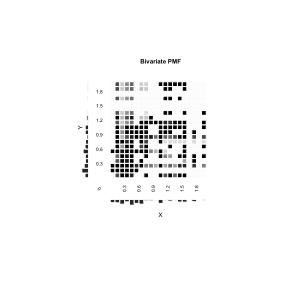The plot above includes the joint probability mass functions (PMFs) displayed as a matrix with darker cells indicating larger probability mass and the marginal PMFs on the left and bottom sides. If we build a proper survival surface by assigning the survival probability to zero after the follow-up time, we get a PDF with the left-over probability ‘tails’ (more noticeable in the marginal PMFs), bivarSurfWithTails = rbind(cbind(dabrSurf, rep(0, nrow(dabrSurf))), rep(0, ncol(dabrSurf)+1)) lastRowVal = as.numeric(rownames(dabrSurf)[nrow(dabrSurf)]) + 2*gridWidth lastColVal = as.numeric(colnames(dabrSurf)[ncol(dabrSurf)]) + 2*gridWidth rownames(bivarSurfWithTails) = c(rownames(dabrSurf), as.character(lastRowVal)) colnames(bivarSurfWithTails) = c(colnames(dabrSurf), as.character(lastColVal)) survPMFPlot(bivarSurf = bivarSurfWithTails, gridWidthX = gridWidth, gridWidthY = gridWidth, scaleGapX = 1.5, scaleGapY = 1.5, XAxisLabel = "X", YAxisLabel = "Y", timeLabelScale = 1, axisLabelScale = 1, labelSkipX = 2, labelSkipY = 2, roundX = 2, roundY = 2, plotLabel = "Bivariate PMF")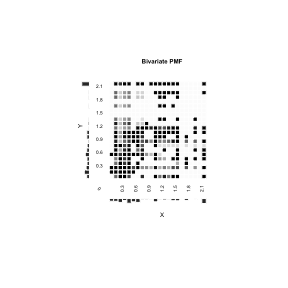Finally, let’s plot a conditional PDF within the restricted region $$[0, 1.4) \times [0, 1.4)$$, condSurf = survRestricted (bivarSurf = dabrSurf, tauX = 1.4, tauY = 1.4)$Sxy
survPMFPlot(bivarSurf = condSurf, gridWidthX = gridWidth, gridWidthY = gridWidth, scaleGapX = 1.5, scaleGapY = 1.5, XAxisLabel = "X", YAxisLabel = "Y", timeLabelScale = 1, axisLabelScale = 1, labelSkipX = 2, labelSkipY = 2, roundX = 2, roundY = 2, plotLabel = "Bivariate PMF")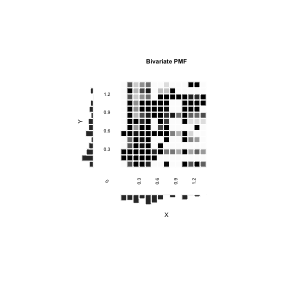## Miscellaneous functions

Function survPMFPlot() provides only basic visualization tools. If users want to plot joint and/or marginal PMFs using their own format, they can take advantage of the following miscellaneous functions.

### Function plotVector()

Function plotVector() plots a vector of numeric values as a set of rectangles with user-specified height, width, color, and border color. The bases of the rectangles are aligned like in a histogram,

### Histogram-like plot
plot(c(-0.5, 1.5), c(-0.5, 1), type = "n", xlab = "", ylab = "", cex.axis = 0.5)
vectValues = c(0.057, 0.336, 0.260, 0.362, 0.209)
plotVector(vectValues, width = 0.2, coordX = 0, coordY = 0, rotationRadians = 0,
vectColor = "gray", bordColor = "white")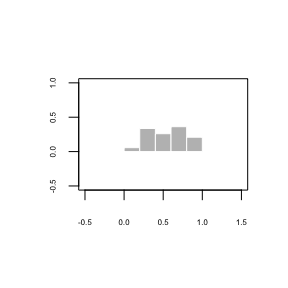These histogram-like plots can be rotated by changing argument rotationRadians,

width = c(0.10, 0.20, 0.10, 0.80, 0.12)
vectColor = c("orange", "green", "orchid", "blue", "goldenrod1")
plot(c(-0.5, 1.5), c(-0.5, 1.5), type = "n", xlab = "", ylab = "", cex.axis = 0.5)
plotVector(vectValues = vectValues, width, coordX = 0, coordY = 0,
rotationRadians = pi/2, vectColor, bordColor = "white")
plotVector(vectValues = vectValues, width, coordX = 0, coordY = 0,
rotationRadians = pi/4, vectColor, bordColor = "white")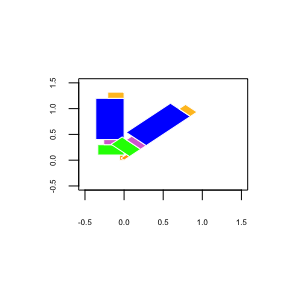and/or flipped by setting argument vectValues to negative values,

width = c(0.10, 0.20, 0.10, 0.80, 0.12)
vectColor = c("orange", "green", "orchid", "blue", "goldenrod1")
plot(c(-0.5, 1.5), c(-0.5, 1.5), type = "n", xlab = "", ylab = "", cex.axis = 0.5)
plotVector(vectValues = vectValues, width, coordX = 0, coordY = 0,
rotationRadians = pi/2, vectColor, bordColor = "white")
plotVector(vectValues = -vectValues, width, coordX = 0, coordY = 0,
rotationRadians = pi/2, vectColor, bordColor = "white")
plotVector(vectValues = -vectValues, width, coordX = 0, coordY = 0,
rotationRadians = pi/4, vectColor, bordColor = "white")
plotVector(vectValues = -vectValues, width, coordX = 0, coordY = 0,
rotationRadians = 0, vectColor, bordColor = "white")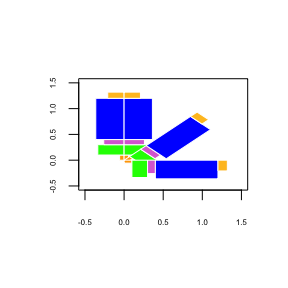Mixing positive and negative values in argument vectValues will result in the following:

vectValues = c(0.057, -0.336, 0.260, -0.222, 0.209)
plot(c(-1, 1), c(-0.5, 0.5), type = "n", xlab = "", ylab = "", cex.axis = 0.5)
vectColor = rep("goldenrod1", length(vectValues))
vectColor[vectValues<0] = "royalblue1"
bordColor = rep("red", length(vectValues))
bordColor[vectValues<0] = "darkblue"
plotVector(vectValues, width = 0.4, coordX = -1, coordY = 0, rotationRadians = 0,
vectColor, bordColor = bordColor)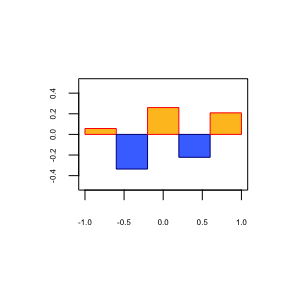### Function plotMatrix()

Function plotMatrix() Plots a matrix of colors at given coordinates. Each element of the color matrix is plotted as a rectangle with user-specified side lengths and border color,

colorMatr = matrix(c("goldenrod1", "mediumpurple3", "palegreen3",
"royalblue1", "orchid", "firebrick1"), nrow = 2, byrow = TRUE)
plot(c(1, 4), c(2, 4), type = "n", xlab = "", ylab = "", cex.axis = 0.5)
polygon(x = c(0, 5, 5, 0, 0), y = c(0, 0, 5, 5, 0), col = "black")
plotMatrix(colorMatr, borderCol = "black", coordX = 1, coordY = 2, widthX = 1, widthY = 1)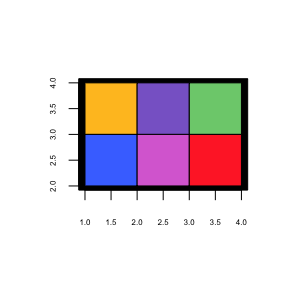The length and width of the rectangles can be different,

colorMatr = matrix(c("goldenrod1", "mediumpurple3", "palegreen3",
"royalblue1", "orchid", "firebrick1"), nrow = 2, byrow = TRUE)
plot(c(1, 4.5), c(2, 4), type = "n", xlab = "", ylab = "", cex.axis = 0.5)
plotMatrix(colorMatr, borderCol = "white", coordX = 1, coordY = 2,
widthX = c(1/4, 1, 1/6), widthY = c(1, 1/2))
reshapeColMatr = matrix(t(colorMatr), ncol = 1, nrow = nrow(colorMatr)*ncol(colorMatr),
byrow = TRUE)
plotMatrix(reshapeColMatr, borderCol = "white", coordX = 2.8, coordY = 2,
widthX = c(1/4), widthY = c(1/4))
text(x = rep(3.03, nrow(reshapeColMatr)),
y = 2.12+c(0,cumsum(rep(1/4, nrow(reshapeColMatr)-1))),
labels = reshapeColMatr[nrow(reshapeColMatr):1], pos = 4, cex = 0.5)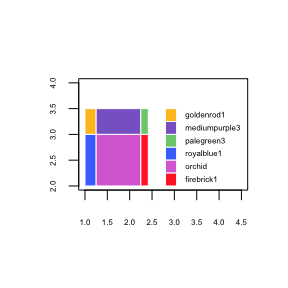### Function fillUpStr()

Function fillUpStr() adds characters to the head or tail of each element of the character vector to make all elements the same length,

fillUpStr(c("A", "aaaa", NA, "bb", "4.5"), where="tail", fill = "-")
#>  "A---" "aaaa" NA     "bb--" "4.5-"

The user can also choose the desired length by setting argument howManyToFill to a value greater than the longest character in the vector,

fillUpStr(c("A", "aaaa", NA, "bb", "4.5"), where="tail", howManyToFill = 10, fill = "-")
#>  "A---------" "aaaa------" NA           "bb--------" "4.5-------"
fillUpStr(c("!", "####", NA, "<<", "4.5"), where="head", howManyToFill = 10, fill = "*")
#>  "*********!" "******####" NA           "********<<" "*******4.5"

### Function fillUpDecimals()

Function fillUpDecimals() formats numbers by adding zeros (or user-specified characters) after the decimal point of each element of a numeric vector so that all elements have the same number of digits after the decimal point,

fillUpDecimals(c("2", "3.4", "A", NA))
#>  "2.0" "3.4" "A.0" NA
fillUpDecimals(c("2", "3.4", "A.5", NA), howManyToFill = 5)
#>  "2.00000" "3.40000" "A.50000" NA
fillUpDecimals(c("2", "3.4", "A.xyz", NA), fill = "?")
#>  "2.???" "3.4??" "A.xyz" NA

If howManyToFill is negative, the function will add . to each non-missing element,

fillUpDecimals(c("2", "3.4", "A", NA), howManyToFill = -3)
#>  "2."  "3.4" "A."  NA

It is not recommended to include characters other than digits or letters into numVec because the function will not work as described for these characters,

fillUpDecimals(numVec = c("2", "3.4", "A.zx", NA, "#", "#a"), fill = "?")
#>  "2.??" "3.4?" "A.zx" NA     "#?"   "#a"